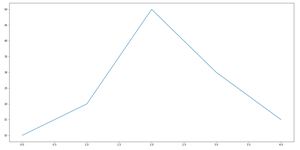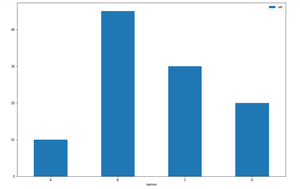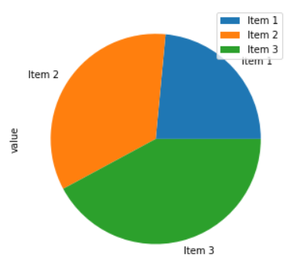Related Articles
Change figure size in Pandas – Python
• Difficulty Level : Easy
• Last Updated : 11 Dec, 2020

Prerequisites: Pandas

The size of a plot can be modified by passing required dimensions as a tuple to the figsize parameter of the plot() method. it is used to determine the size of a figure object.

Syntax:

`figsize=(width, height)`

Where dimensions should be given in inches.

### Approach

• Import pandas.
• Call the plot() function with a figsize parameter along with dimensions.

Example 1

## Python3

 `import` `pandas as pd  ``# import the pandas module`` ` `# python list of numbers``data1 ``=` `[``10``, ``20``, ``50``, ``30``, ``15``]`` ` `# convert the list to a pandas series``s1 ``=` `pd.Series(data1)  `` ` `# creates a figure of size 20 inches wide and 10 inches high``s1.plot(figsize``=``(``20``, ``10``))`

Output:Example 2

## Python3

 `# import the pandas module``import` `pandas as pd  `` ` `# Creating a pandas dataframe``df ``=` `pd.DataFrame({``'names'``: [``'A'``, ``'B'``, ``'C'``, ``'D'``], ``'val'``: [``10``, ``45``, ``30``, ``20``]})`` ` `# creates a bar graph of size 15 inches wide and 10 inches high``df.plot.bar(x``=``'names'``, y``=``'val'``, rot``=``0``, figsize``=``(``15``, ``10``))`

Output :Example 3

## Python3

 `# import the pandas module``import` `pandas as pd`` ` `# Creating a pandas dataframe with index``df ``=` `pd.DataFrame({``'value'``: [``3.330``, ``4.87``, ``5.97``]},``                  ``index``=``[``'Item 1'``, ``'Item 2'``, ``'Item 3'``])`` ` `df.plot.pie(y``=``'value'``, figsize``=``(``5``, ``5``))`

Output :Attention geek! Strengthen your foundations with the Python Programming Foundation Course and learn the basics.

To begin with, your interview preparations Enhance your Data Structures concepts with the Python DS Course. And to begin with your Machine Learning Journey, join the Machine Learning – Basic Level Course

My Personal Notes arrow_drop_up